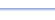Program: FACTOR

FACTOR

• Finds all factors of a number.
• Finds the prime factorization of a number.

Useful for:

• SAT
• ACT
• Basic Math
• Algebra 1
• Algebra 2

See full list of programsExample 1

Q: Find the positive factors of 22.

Solution: Run FACTOR.  How?

Choose '2' to find
all factors.

Enter 22 for the
number.

The factors will
appear two at a
time, as pairs of
numbers that
multiply to 22.

Press ENTER to see more factors.  Keep pressing ENTER until the program says 'Done'.  **If the list is too long, the numbers may scroll off the top of the screen!**  That is why the numbers appear two at a time - so that you can write them down incase the list is too long.

Interpretation:  The factors of 22 are: 1, 2, 11, and 22.

*Tip: Use FACTOR to determine if a number is prime.  If the program reports only 1 and the number itself before saying 'Done', then the number is prime.

See Example 1
See Example 2